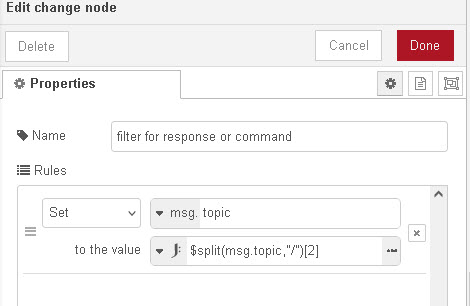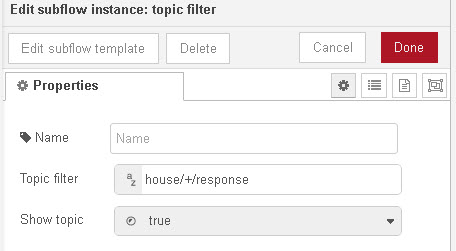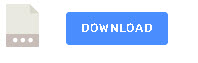# Filtering MQTT Topics (commands and Responses) in Node-Red

Many MQTT topics have  control structure, for example, to turn a Tasmota switch on/off you use a topic with an embedded command and value e.g

house/main-light/command/power/on

where

• house is a topic prefix
• main-light is the ID of the switch
• Command specifies a command
• power is the attribute of the device
• on is the value

the switch will respond with the topic

house/main-light/stat/result

• house is a topic prefix
• main-light is the ID of the switch
• stat -status
• result -result of command

When monitoring these commands and responses in a node-red flow the first job is to decide how we will subscribe to the topic.

Generally we will subscribe using a wild card like this

house/#

and then filter the topics.

We could also subscribe using 2 MQTT nodes on topics:

house/+/command
house/+/response

We now have separate streams for commands and responses.

Depending on the topic structure we may need to apply multiple filters.

#### Using the Function node and Split

The most common method is to use a function node to filter the topics. The first step is usually to spit the topics.

`topics=msg.topic.split("/");`

will give us an array containing the topic parts. To access the command we use:

`let command= topics;`

We can know check if this is valid using a simple if statement.

```if(command=="command" || command="cmnd")
//we have a command```

we can do a similar thing for the response:

`let response=topics;`

We can now check if this is valid using a simple if statement.

```if(command=="stat" || command="response")
//we have a response```

Sample code for the function node is shown below:

```let topic=msg.topic;
topic=topic.split("/");
if (topic=="response" || topic=="RESPONSE")
{
return[null,msg];
}
if (topic == "command" || topic== "COMMAND" || topic== "cmnd" )
{
return[msg,null]
}```

### Using JSONata

You can also filter using the change node with JSONata as shown below:JSONata is very powerful but I find using the function node and JavaScript easier

#### Using Topic Filter Sub Flow

The  topic filter sub flow makes topic filtering easy as you just enter the filter pattern in the node.Because you will probably need to do filtering in several flows it is probably wise to use a sub flow.

If you take a look at the flow you will find that it uses function nodes to do the filtering.

#### Demo Flow

The example flow demonstrates various filter methods that you can use:Related Tutorials and resources:

Click to rate this post!
[Total: 0 Average: 0]